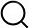# Also in the Article

2.6. Comparison metrics

Procedure

To assess the sensitivity of a given parameter of the framework, we compared connectome harmonics by computing Pearson correlations between the harmonic patterns emerging from unaltered and altered connectomes. We also compared each harmonic to the DMN using mutual information as in (Atasoy et al., 2016). These metrics serve different purposes: Pearson correlation permits quantification of similarity between harmonics, while mutual information indirectly measures the structure-function relationships between each harmonic and the DMN.

Each connectome harmonic is represented by a vector of the size of the number of vertices in a cortical surface mesh. When smoothing the cortical surface mesh or changing its resolution, the ordering of vertices is affected. To overcome such disorganization, the harmonic vectors are projected onto the coarser space of the Desikan-Killiany atlas, which is conserved by region mapping. We refer to this coarser atlas space as the lower resolution alternative to the higher resolution mesh space. The similarity between harmonics of two different meshes is then assessed in the lower resolution atlas space by computing the correlation P between the two coarse harmonic vectors. As two sets of connectome harmonics become identical, a very high correlation value results (P → 1) for pairs of harmonics with the same index, results in a diagonal harmonics correlation matrix (Fig. 6, ,77 and and8).8). Divergence from the diagonal matrix reflects a decrease in correlation between harmonics of the same index across the two sets, reflecting a disorganization of the eigen-decomposition output in the form of eigenvalue/eigenvector pairs.

Low frequency harmonics are robust to cortical surface changes. High-resolution connectome and connectome harmonics are recomputed for different smoothing levels of WMGM cortical surface (left), or pial surface (right) and compared to original WMGM surface mesh using Pearson correlation in atlas space for the first 100 harmonics. Adjacency weight threshold is set to $zC=1$, corresponding to a proportion r ≃ 0.7 of local connections.

Low frequency harmonics are robust to mesh resolution changes. Region-wise correlation of connectome harmonics $ψk∈K={1,…,100}$ using fsaverage4 (5,124 vertices) and fsaverage5 (20,484 vertices) cortical surface mesh templates from FreeSurfer. Proportion r of local connections is indicated by different degrees of connectome adjacency weight threshold $zC$. Insets show a magnified version of the correlation matrix for connectome harmonics $ψk∈K={1,…,20}$.

The Mutual Information (MI) between a connectome harmonic ψk and the DMN vector vDMN was computed as follows:

where p(n) is the probability distribution of n, and p(n, m) is the joint probability distribution of n and m, with vDMN being the vector representing the Default Mode Network in atlas space. The values of the harmonic vector were discretized into N=16 bins, while the DMN is represented by a binary vector (M=2 bins), whether the cortical mesh vertex is part of the DMN or not (Atasoy et al., 2016). Mapping of the Default Mode Network (DMN) to the Desikan-Killiany atlas is provided in Suppl. Table 1.

Q&A